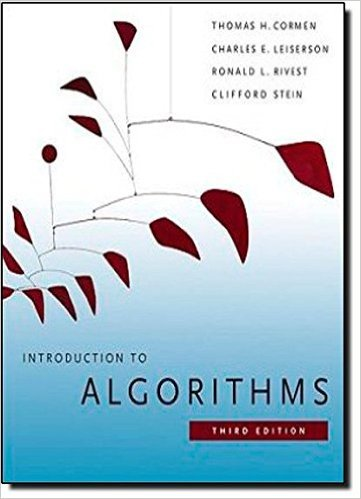×
Get Full Access to Introduction To Algorithms - 3 Edition - Chapter 2 - Problem 2-1
Get Full Access to Introduction To Algorithms - 3 Edition - Chapter 2 - Problem 2-1

×

# Insertion sort on small arrays in merge sort AlthoughISBN: 9780262033848 130

## Solution for problem 2-1 Chapter 2

Introduction to Algorithms | 3rd Edition

• Textbook Solutions
• 2901 Step-by-step solutions solved by professors and subject experts
• Get 24/7 help from StudySoup virtual teaching assistantsIntroduction to Algorithms | 3rd Edition

4 5 1 353 Reviews
20
3
Problem 2-1

Insertion sort on small arrays in merge sort Although merge sort runs in .n lg n/ worst-case time and insertion sort runs in .n2/ worst-case time, the constant factors in insertion sort can make it faster in practice for small problem sizes on many machines. Thus, it makes sense to coarsen the leaves of the recursion by using insertion sort within merge sort when subproblems become sufficiently small. Consider a modification to merge sort in which n=k sublists of length k are sorted using insertion sort and then merged using the standard merging mechanism, where k is a value to be determined. a. Show that insertion sort can sort the n=k sublists, each of length k, in .nk/ worst-case time. b. Show how to merge the sublists in .n lg.n=k// worst-case time. c. Given that the modified algorithm runs in .nk C n lg.n=k// worst-case time, what is the largest value of k as a function of n for which the modified algorithm has the same running time as standard merge sort, in terms of -notation? d. How should we choose k in practice?

Step-by-Step Solution:
Step 1 of 3

Thursday, January 12, 2017 2:06 PM 13.4 Page 1 13.4 Page 2

Step 2 of 3

Step 3 of 3

##### ISBN: 9780262033848

The full step-by-step solution to problem: 2-1 from chapter: 2 was answered by , our top Engineering and Tech solution expert on 11/10/17, 05:55PM. Since the solution to 2-1 from 2 chapter was answered, more than 287 students have viewed the full step-by-step answer. Introduction to Algorithms was written by and is associated to the ISBN: 9780262033848. The answer to “Insertion sort on small arrays in merge sort Although merge sort runs in .n lg n/ worst-case time and insertion sort runs in .n2/ worst-case time, the constant factors in insertion sort can make it faster in practice for small problem sizes on many machines. Thus, it makes sense to coarsen the leaves of the recursion by using insertion sort within merge sort when subproblems become sufficiently small. Consider a modification to merge sort in which n=k sublists of length k are sorted using insertion sort and then merged using the standard merging mechanism, where k is a value to be determined. a. Show that insertion sort can sort the n=k sublists, each of length k, in .nk/ worst-case time. b. Show how to merge the sublists in .n lg.n=k// worst-case time. c. Given that the modified algorithm runs in .nk C n lg.n=k// worst-case time, what is the largest value of k as a function of n for which the modified algorithm has the same running time as standard merge sort, in terms of -notation? d. How should we choose k in practice?” is broken down into a number of easy to follow steps, and 184 words. This textbook survival guide was created for the textbook: Introduction to Algorithms, edition: 3. This full solution covers the following key subjects: sort, Insertion, merge, worst, Case. This expansive textbook survival guide covers 35 chapters, and 151 solutions.

Unlock Textbook Solution